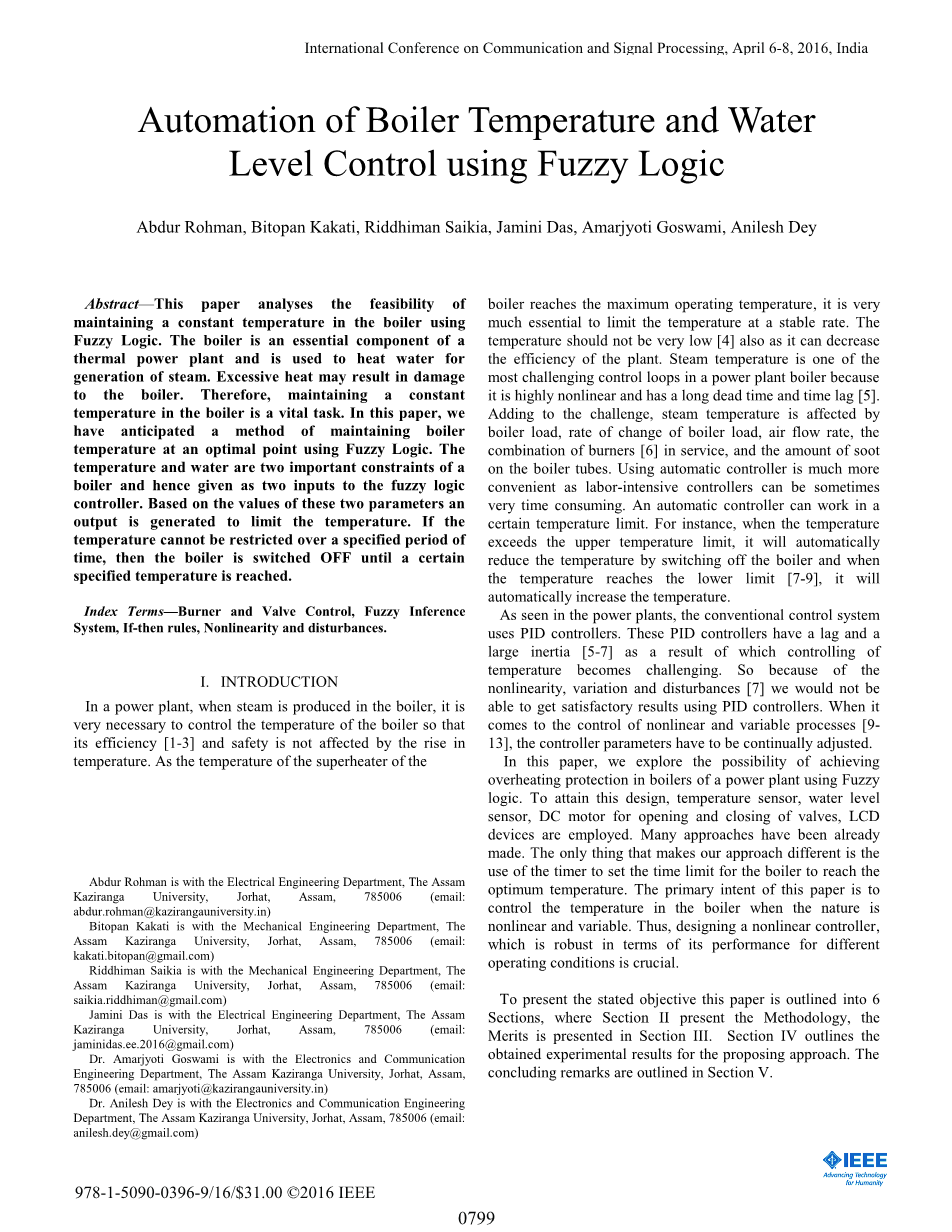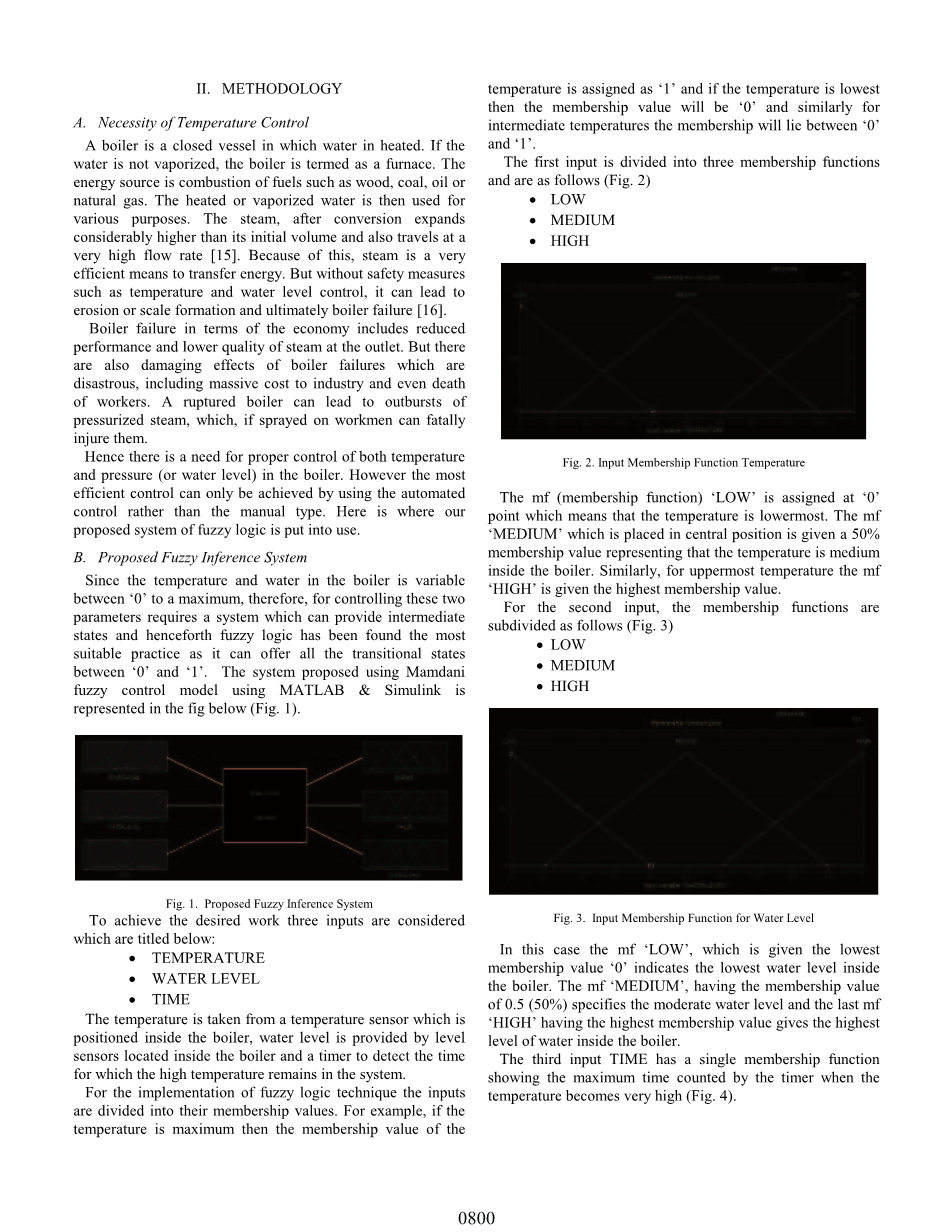# 基于模糊理论的锅炉温度和水位控制自动化外文翻译资料

2021-11-26 10:11# Abdur Rohman, Bitopan Kakati, Riddhiman Saikia, Jamini Das, Amarjyoti Goswami, Anilesh Dey

1. 介绍

Abdur Rohman在阿萨姆卡齐兰加大学电气工程系，Jorhat，Assam，785006（电子邮件：abdur.rohman@kazirangauniversity.in）

Bitopan Kakati是机械工程系，阿萨姆卡齐兰加大学，Jorhat，Assam，785006（电子邮件：kakati.bitopan@gmail.com）

Riddhiman Saikia是机械工程系，阿萨姆卡齐兰加大学，Jorhat，Assam，785006（电子邮件：saikia.riddhiman@gmail.com）

Jamini Das是电气工程系，阿萨姆卡齐兰加大学，Jorhat，Assam，785006（电子邮件：jaminidas.ee.2016@gmail.com）

Amarjyoti Goswami博士是电子与通信工程系，阿萨姆卡齐兰加大学，Jorhat，Assam，785006（电子邮件：amarjyoti@kazirangauniversity.in）

Anilesh Dey博士是电子与通信工程系，阿萨姆卡齐兰加大学，Jorhat，Assam，785006（电子邮件：anilesh.dey@gmail.com）

# 978-1-5090-0396-9/16/\$31.00 copy;2016 IEEE

1. 方法

A.温度控制的必要性

B.Proposed模糊推理系统

bull;温度

bull;水位

bull;时间

mf（成员函数）#39;低#39;在#39;0#39;点分配，这意味着温度最低。 位于中心位置的mf#39;中#39;具有50％的隶属度值，表示锅炉内的温度中等。 类似地，对于最高温度，mf“高”被赋予最高的成员值。

• 燃烧器
• 活塞
• 系统闭阀

• 全开

A.建议的控制系统

• 如果温度和水位都设置为HIGH，则燃烧器和阀门都设置为OFF。
• 如果温度中等且水位低，则燃烧器设置为低，阀门设置为HIGH，因为我们需要更多蒸汽。
• 如果温度低且水位高，则燃烧器为高电平且阀门关闭。

1. 优点

1. 结果与讨论

F(x, y) = p00 p10*x p01*y p20*x^2 p11*x*y p02*y^2 p30*x^3 p21*x^2*y p12*x*y^2 p03*y^3 (1)

p00 = 0.9115 (0.8781, 0.945)

p10 = -1.809 (-1.988, -1.631)

p01 = -0.03168 (-0.21, 0.1467)

p20 = 2.959 (2.603, 3.314)

p11 = 0.06336 (-0.2158, 0.3426)

p02 = 0.03168 (-0.3241, 0.3875)

p30 = -1.972 (-2.197, -1.748)

p21 = -5.807e-016 (-0.1938, 0.1938)

p12 = -0.06336 (-0.2572, 0.1305)

p03 = 2.013e-015 (-0.2247, 0.2247)

F(x, y) = p00 p10*x p01*y p20*x^2 p11*x*y p02*y^2 p30*x^3 p21*x^2*y p12*x*y^2 p03*y^3 p40*x^4 p31*x^3*y p22*x^2*y^2 p13*x*y^3 p04*y^4 p50*x^5 p41*x^4*y p32*x^3*y^2 p23*x^2*y^3 p14*x*y^4 p05*y^5 (2)

lt;